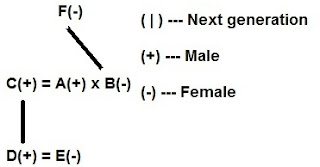## Reasoning Notes: Blood Relations

BLOOD RELATIONS - NOTES
Important Points to Remember
a) While attempting Blood Relation questions, first we should read the ‘Directions’ given carefully
b) Then pin-point the two persons between whom the relationship is to be established
c) Try to connect the given relations
d) While determining the relationships between two persons one should be careful about the sexes of the persons involved
e) Sometimes it is not possible to define relationships between two persons without knowing their sex
1. Relations of Paternal side:
Father's father → Grandfather
Father's mother → Grandmother
Father's brother → Uncle
Father's sister → Aunt
Children of uncle → Cousin
Wife of uncle → Aunt
Children of aunt → Cousin
Husband of aunt → Uncle
2. Relations of Maternal side:
Mother's father → Maternal grandfather
Mother's mother → Maternal grandmother
Mother's brother Maternal uncle
Mother's sister → Aunt
Children of maternal uncle → Cousin
Wife of maternal uncle → Maternal aunt
Relations from one generation to next:Different types of questions with explanation:
Type 1: Single Person Blood Relations or Descriptive type
1. Introducing a boy, a girl said, "He is the son of the daughter of the father of my uncle." How is the boy related to the girl?
Solution:
The father of the boy's uncle => the grandfather of the boy and daughter of the grandfather => sister of father
2. Pointing to a photograph, Vipul said, "She is the daughter of my grandfather's only son." How is Vipul related to the girl in the photograph?
Solution:
My grandfather's only son => My father
So, the girl is the daughter of Vipul's father i.e., Vipul is the girl`s brother.
3. A girl introduced a boy as the son of the daughter of the father of her uncle. The boy is girl`s
Solution:
Daughter of uncle's father => Uncle's sister => Mother;
Mother's son => Brother
Type 2: Coded type relations
Directions for questions (4 - 5): Answer the questions based on the following information given below:
I. A \$ B means A is mother of B.
II. A # B means A is father of B.
III. A @ B means A is husband of B.
IV. A % B means A is daughter of B.
4. P @ Q \$ M # T indicates what relationship P with T?
Solution:
P @ Q \$ M # T means
P is the husband of Q and Q is the mother of M and M is the father of T. so, clearly P is T’s paternal grandfather.
5. If G \$ M @ K, how is K related to G?
Solution:
G \$ M @ K means
G is the mother of M and M is the husband of K. Clearly K is G’s daughter-in la
6. U + V means U is the brother of V,
W – X means W is the father of S,
X  Y means X is the sister of Y,
Y  Z means Z is the mother of Y.
Which of the following means that N is the mother of O?
a) L + M ÷ N × O
b) L – M × O ÷ P
c) N ÷ M × L ÷ O
d) M + L ÷ O × N
Solution:
M + L ÷ O
N means M is the brother of L and L is the sister of O and N is the mother of O.
Type 3: Puzzle Type relations
7. T is son of P, S is son of Q, T is married to R, R is Q’s daughter, U is son of S. How U is related to R.
Solution:U is Son of R’s brother. Then U is nephew of R
Directions (8 - 10): Read the following information carefully and answer the questions given below it:
In a family, there are six members A, B, C, D, E and F. A and B are a married couple, A being the male member. D is the only son of C, who is the brother of A. E is the sister of D. B is the daughter-in-law of F, whose husband has died.
Explanation:8. How is F related to A?
Solution:
F is Mother of A
Type 4: Data Sufficiency type relations
9. How is T related to K?
Statements:
I. R's sister J has married Ts brother L, who is the only son of his parents.
II. K is the only daughter of L and J.
Solution:
From I, we know that L is T's brother and J's husband. Since L is the only son of his parents, T is L's sister.
From II, we know that K is L's daughter.
Thus, from I and II, we conclude that T is the sister of K's father i.e. T is K's aunt.
10. How many children does M have ?
Statements:
I. H is the only daughter of X who is wife of M.
II. K and J are brothers of M.
Solution:
From I, we conclude that H is the only daughter of M. But this does not indicate that M has no son. The information given in II is immaterial.

 Set – 2 Set – 3 Set – 4 Set – 5 Set – 6 Set – 7 Set – 8 Set – 9 Set – 10